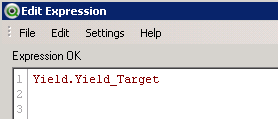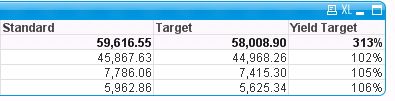# QlikView App Dev

Discussion Board for collaboration related to QlikView App Development.

Announcements
Skip the ticket, Chat with Qlik Support instead for instant assistance.
cancel
Showing results for
Did you mean:Creator

## Straight table calculated total row

Hi All,

My requirement is to have a calculated total different to it's expression.

My expression is simply calling a field and I want the total of this column to be a calculation of two previously calculated expressions.The Yield target is not a calculated so the total is not correct as can be seen as blank below.I would like the Yield Target total to be Standard / Target so it would be 59616.55 / 58008.90

I should also point out that the standard and target columns are both expressions.

Thanks,

Tom

1 Solution

Accepted SolutionsMVP

try this expression

SUM(DISTINCT [Target Content])/

(sum(aggr(sum([Target Content])/sum(Yield_Target),Resource,Date)))

15 RepliesAnonymous
Not applicable

Neal, didn't this work [Standard]/[Target]??MVP

Should be this for Yield Target

Sum(TOTAL [Standard]) / Sum(TOTAL [Target])

Before develop something, think If placed (The Right information | To the right people | At the Right time | In the Right place | With the Right context)Creator
Author

Unfortunately not,  unless I've overlooked something the result I got for Standard/Target is 313% as the Total.

That calculation works for the row values but not the total.  The result I should get in the total is 102.7%MVP

Seems Working?Before develop something, think If placed (The Right information | To the right people | At the Right time | In the Right place | With the Right context)Creator
Author

The Standard and Target columns are expressions.

This is my expression....

Standard = SUM(DISTINCT  [Yield.Standard])

Target        = sum(TOTAL [Yield.Standard])/sum(TOTAL Yield.Yield_Target)

Yield_Target =

SUM(DISTINCT  [Yield.Standard])/
(
sum([Yield.Standard])/sum(Yield.Yield_Target))

The result is belowMVP

Is there any dimensions in your report, Would you able to provide qvw for testing

Before develop something, think If placed (The Right information | To the right people | At the Right time | In the Right place | With the Right context)Anonymous
Not applicable

Use the columns in the straight table. You can reference earlier columns in the table,Creator
Author

How can I reference expression columns in a straight table?Creator
Author

Yes here is a test qvw.Community Browser